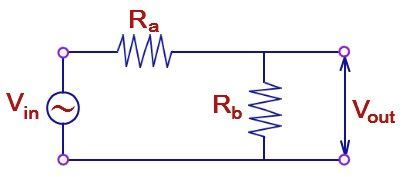# Voltage Divider Formula

The voltage divider is the series of resistors or capacitors that can be tapped at any intermediate point to generate a specific fraction of the voltage applied between its ends.

It consists of an electric circuit composed of two resistors and one input voltage supply. The below figure shows a simple voltage divider. In this circuit, two resistors are connected in series. The output voltage of the voltage divider is a function of the input voltage. This circuit helps to determine how the input voltage divides among the components in the circuit.The voltage divider formula is given by,

Where,

• Voutis the output voltage
• Ra and Rb are the resistors
• Vin is the input voltage

### Solved Examples

Example 1

Determine the output voltage of the voltage divider circuit whose Ra and Rb are 6 Ω and 8 Ω respectively and the input voltage is 10v.

Solution:

Given:

Ra = 6Ω,

Rb = 8Ω

Vin = 10V

Voltage divider formula is given by,

= [8 / (6 + 8)] 10

Vout = 5.71V

Example 2

The value of the input voltage of a voltage divider is 20V, and the resistors are 5 Ω and 7 Ω. Determine the output voltage.

Solution:

Given:

Ra = 5 Ω

Rb = 7 Ω

Vin = 20V

Voltage divider formula is given by,

=[ 7 /( 5 + 7 )]20

Vout = 11.66V

For more such valuable equations and formulas stay tuned with BYJU’S!!